# characteristic

(redirected from anal characteristic)
Also found in: Dictionary, Thesaurus, Medical.

## characteristic

Maths
a. the integral part of a common logarithm, indicating the order of magnitude of the associated number
b. another name for exponent esp in number representation in computing
Collins Discovery Encyclopedia, 1st edition © HarperCollins Publishers 2005
The following article is from The Great Soviet Encyclopedia (1979). It might be outdated or ideologically biased.

## Characteristic

in mathematics. (1) The greatest integer in a common logarithm.

(2) A concept of the theory of partial differential equations. In the case of a differential equation of the first order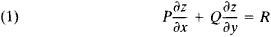where P = P(x, y, z), Q = Q(x, y, z), and R = R(x, y, z) are given functions, the characteristics of the equation are defined as the curves determined by the system of differential equations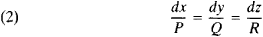Integrating the system (2), we obtain the family of characteristics ϕ(x, y, z) = C1, ψ(x, y, z) = C2, where C1 and C2 are arbitrary constants. This family is the set of curves tangent at each of their points to the vector {P, Q, R}.

Each integral surface of equation (1) is the geometric locus of the characteristics intersecting some curve. The equation of such a surface can be represented in the form F[ϕ(x, y, z), ψ(x, y, z)] = 0, where F is a function of two variables. Conversely, to find the integral surface passing through a given curve, it is sufficient to construct the geometric locus of the characteristics intersecting the curve (seeCAUCHY PROBLEM). The Cauchy problem has one and only one solution if the given curve is not a characteristic.

The concept of a characteristic can be extended to equations of the first order with more than two independent variables.

For an equation of the second order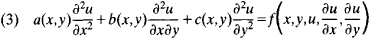the characteristics were introduced by G. Monge in 1784 and 1795 as the curves along which the differential equation

(4) ady2b dx dy + c dx2 = 0

is satisfied. If equation (3) is of hyperbolic type, then there are two families of characteristics whose equations are ξ,(x, y) = C1 and η(x, y) = C2; here, C1 and C2 are arbitrary constants. If we take ξ; and η as new independent variables, then equation (3) reduces to an equation of the form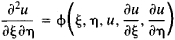The two families of characteristics coincide if equation (3) is of parabolic type. For an arbitrary choice of the independent variable η, equation (3) reduces to an equation of the form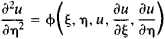If equation (3) is of elliptic type, then it has no real characteristics. If the solution of equation (4) is represented in the form ξ ± iη = C, then equation (3) transforms into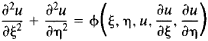The values of a solution u at each point of a characteristic and the values of ∂a/∂x and ∂u/∂y at any one of the points completely determine the values of these derivatives at all points of the characteristic. [This fact underlies the method of characteristics for the solution of boundary value problems for equation (3).] Such a relationship does not exist for curves other than characteristics. On the other hand, the values of u, ∂u/∂x, and ∂u/∂y on a curve that is not a characteristic determine the solution u in a neighborhood of the curve; this relationship does not hold for characteristics. If two solutions of equation (3) coincide on one side of a curve and are different on the other, then the curve is a characteristic.

If the coefficients of equation (3) depend on u, ∂u/∂x, and ∂u/∂y (the quasi-linear case), then the characteristics determined by equation (4) are different for different solutions.

There exist definitions of characteristics for partial differential equations of any order and for systems of such equations.

## Characteristic

in technology, the relationship between a dependent and an independent variable that defines the state of a technological process, instrument, piece of equipment, machine, or system; it may be expressed in the form of a text, table, mathematical formula, or plotted curve. Examples of such relationships are the dependence of current on voltage in a subcircuit, the fuel consumption of a motor vehicle on the distance traveled and the condition of the road, the loudness and quality of sounds produced by a loudspeaker on the frequency of the sounds, and the magnetization time reversal of a ferrite core on the strength of the magnetizing field.

Characteristics may be classified as determinate (static or dynamic) or statistical, depending on the method of measurement; as linear or nonlinear, depending on the type of analytical relationship; and as operational or adjustment, depending on the purpose. The relationship between the output and input values of a technological system under steady-state conditions is called the static characteristic. Dynamic characteristics (frequency, pulse, and others) indicate the reactions of a system under study to certain standard perturbations. For example, the frequency characteristic describes the dependence of the amplitude and phase of a periodic signal at the output of a system on the amplitude and phase of a harmonic input signal when only the frequency is varied; the pulse characteristic describes the dependence of the variation in time of a signal at the output of a system on the action of a single input pulse. A dynamic characteristic is contained in the most complete form in the dynamic mathematical model of an object, for example, in the form of differential equations. A statistical characteristic is suitable for objects whose behavior varies with time in a random fashion. Statistical characteristics include dispersions, autocorrelation functions, and spectral densities.

All characteristics may be termed linear if they can be approximated with some specified accuracy by an expression of the form y = ax + b, where y is the output action, x is the input action on the system under study, and a and b are constants. All other characteristics are called nonlinear, including those that can be approximated in sections by the expression given above with some specified accuracy (seeLINEARIZATION).

A. V. KOCHEROV

## characteristic

[‚kar·ik·tə′ris·tik]
(electronics)
A graph showing how the voltage or current between two terminals of an electronic device varies with the voltage or current between two other terminals.
(mathematics)
That part of the logarithm of a number which is the integral (the whole number) to the left of the decimal point in the logarithm.
For a family of surfaces that depend continuously on a parameter, the limiting curve of intersection of two members of the family as the two values of the parameter determining them approach a common value.
For a ring or field, the smallest possible integer whose product with any element of the ring or field equals zero, provided that such an integer exists; otherwise the characteristic is zero.
McGraw-Hill Dictionary of Scientific & Technical Terms, 6E, Copyright © 2003 by The McGraw-Hill Companies, Inc.

## characteristic

In logarithms and floating point, the number that indicates where the decimal point is placed.
Copyright © 1981-2019 by The Computer Language Company Inc. All Rights reserved. THIS DEFINITION IS FOR PERSONAL USE ONLY. All other reproduction is strictly prohibited without permission from the publisher.
Site: Follow: Share:
Open / Close